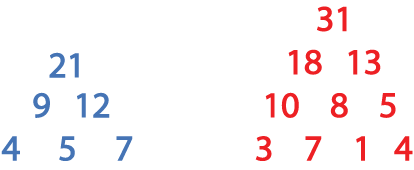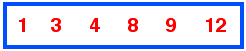#### You may also like### Summing Consecutive Numbers

15 = 7 + 8 and 10 = 1 + 2 + 3 + 4. Can you say which numbers can be expressed as the sum of two or more consecutive integers?### Always the Same

Arrange the numbers 1 to 16 into a 4 by 4 array. Choose a number. Cross out the numbers on the same row and column. Repeat this process. Add up you four numbers. Why do they always add up to 34?### Fibs

The well known Fibonacci sequence is 1 ,1, 2, 3, 5, 8, 13, 21.... How many Fibonacci sequences can you find containing the number 196 as one of the terms?

# Top-heavy Pyramids

##### Age 11 to 14Challenge Level

This problem follows on from More Number Pyramids.

These are top-heavy pyramids. The blue one has $21$ at the apex (top) and the red one has $31$.A pair of numbers are added to make the number above that pair.

In the blue top-heavy pyramid whose base is $4$, $5$ and $7$, $4 + 5 = 9$, so $9$ is placed between and above the $4$ and the $5$.
$5 + 7 = 12$ and $9 + 12 = 21$.

Use the numbers in the box below to make the base of a top-heavy pyramid whose top number is $200$.